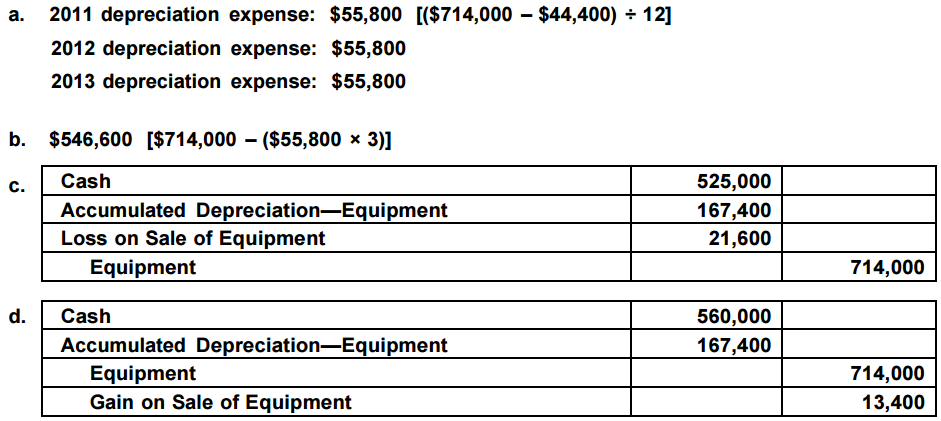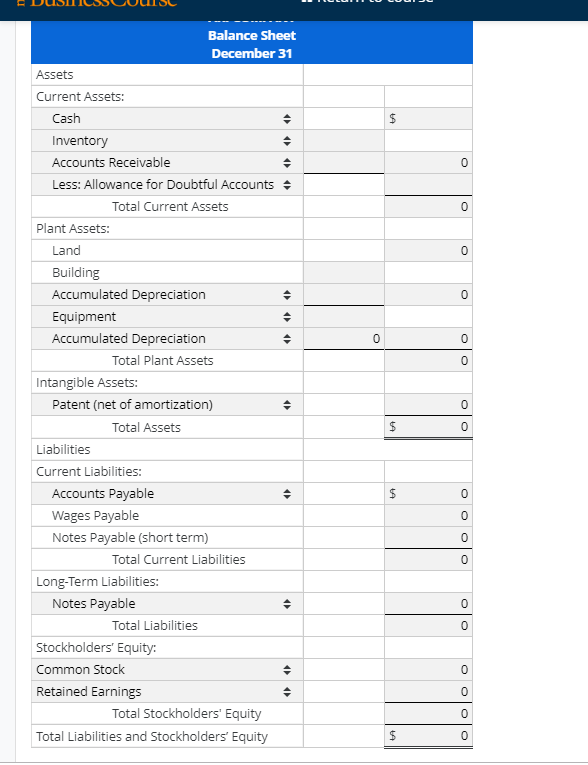# accumulated depreciation

accumulated depreciation

Net book value is the cost of an asset subtracted by its accumulated depreciation. For example, a company purchased a piece of printing equipment for \$100,000 and the accumulated depreciation is \$35,000, then the net book value of the printing equipment is \$65,000. The accumulated depreciation account is a contra asset account on a company’s balance sheet, meaning it has a credit balance. It appears on the balance sheet as a reduction from the gross amount of fixed assets reported.

Accumulated depreciation is the total depreciation expense a business has applied to a fixed asset since its purchase. At the end of an asset’s operating life, its accumulated depreciation equals the price the corporate owner originally paid — assuming the resource’s salvage value is zero.

If not, accumulated depreciation equals the asset’s book value minus its residual worth. “Fixed asset” is what finance people call a tangible asset, capital resource, physical asset or depreciable resource. When recording depreciation in the general ledger, a company debits depreciation expense and credits accumulated depreciation. Depreciation expense flows through to the income statement in the period it is recorded. Accumulated depreciation is presented on the balance sheet below the line for related capitalized assets.

Accumulated depreciation is the total amount a company depreciates its assets, while depreciation expense is the amount a company’s assets are depreciated for a single period. Essentially, accumulated depreciation is the total amount of a company’s cost that has been allocated to depreciation expense since the asset was put into use. When the assets are eventually retired or sold, the accumulated depreciation amount on a company’s balance sheet is reversed, removing the assets from the financial statements. Depreciation spreads the expense of a fixed asset over the years of the estimated useful life of the asset.

The accumulated depreciation balance increases over time, adding the amount of depreciation expense recorded in the current period. Accumulated depreciation is the total amount an asset has been depreciated up until a single point. Each period, the depreciation expense recorded in that period is added to the beginning accumulated depreciation balance. An asset’s carrying value on the balance sheet is the difference between its historical cost and accumulated depreciation. At the end of an asset’s useful life, its carrying value on the balance sheet will match its salvage value.

The accounting entries for depreciation are a debit to depreciation expense and a credit to fixed asset depreciation accumulation. Each recording of depreciation expense increases the depreciation cost balance and decreases the value of the asset.

### Is accumulated depreciation equipment an asset?

Accumulated depreciation – equipment is the aggregate amount of depreciation that has been charged against the equipment asset. The balance in this account is paired with the equipment fixed asset account to arrive at the net book value of all equipment.

The method of accounting used to allocate the cost of a tangible asset over its useful life and is used to account for declines in value is called depreciation. Business can deduct the cost of the tangible asset they purchase off their taxes but how and when the company can deduct depreciation is dictated by IRS rules. As an example, a company acquires a machine that costs \$60,000, and which has a useful life of five years. This means that it must depreciate the machine at the rate of \$1,000 per month.

## Is Accumulated Depreciation a Current Asset or Fixed Asset?

Depreciation is a method used to allocate the cost of tangible assets or fixed assets over the assets’ useful life. In other words, it allocates a portion of that cost to periods in which the tangible assets helped generate revenues or sales. By charting the decrease in the value of an asset or assets, depreciation reduces the amount of taxes a company or business pays via tax deductions. Over time, the accumulated depreciation balance will continue to increase as more depreciation is added to it, until such time as it equals the original cost of the asset.## Optional cookies and other technologies

Over time, the amount of accumulated depreciation will increase as more depreciation is charged against the fixed assets, resulting in an even lower remaining book value. In using the declining balance method, a company reports larger depreciation expenses during the earlier years of an asset’s useful life. Accumulated depreciation is used in calculating an asset’s net book value.

Companies must be careful in choosing appropriate depreciation methodologies that will accurately represent the asset’s value and expense recognition. Depreciation is found on the income statement, balance sheet, and cash flow statement. It can thus have a big impact on a company’s financial performance overall. The total amount of depreciation expense is recognized as accumulated depreciation on a company’s balance sheet and subtracts from the gross amount of fixed assets reported. The amount of accumulated depreciation increases over time as monthly depreciation expenses are charged against a company’s assets.

• For accounting purposes, the depreciation expense is debited, and the accumulated depreciation is credited.
• Depreciation expenses, on the other hand, are the allocated portion of the cost of a company’s fixed assets that are appropriate for the period.
• Depreciation expense is recognized on the income statement as a non-cash expense that reduces the company’s net income.

The cost for each year you own the asset becomes a business expense for that year. This expense is tax-deductible, so it reduces your business taxable income for the year. To spread the cost of a capital asset, a corporate bookkeeper debits the depreciation expense account and credits the accumulated depreciation account. The last item is a contra-asset account that reduces the worth of the corresponding fixed resource. The accumulated depreciation lies right underneath the “property, plant and equipment” account in a statement of financial position, also known as a balance sheet or report on financial condition.

## AccountingTools

At that time, stop recording any depreciation expense, since the cost of the asset has now been reduced to zero. Accumulated depreciation is the total decrease in the value of an asset on the balance sheet of a business, over time.

## Depreciation

Depreciation expense flows through an income statement, and this is where accumulated depreciation connects to a statement of profit and loss — the other name for an income statement or P&L. The amount of accumulated depreciation for an asset or group of assets will increase over time as depreciation expenses continue to be credited against the assets.

Because a fixed asset does not hold its value over time (like cash does), it needs the carrying value to be gradually reduced. Depreciation expense gradually writes down the value of a fixed asset so that asset values are appropriately represented on the balance sheet. Why would a business willingly choose costlier early expenses on the asset? Accumulated depreciation has a credit balance, because it aggregates the amount of depreciation expense charged against a fixed asset. This account is paired with the fixed assets line item on the balance sheet, so that the combined total of the two accounts reveals the remaining book value of the fixed assets.

## Understanding Accumulated DepreciationWhen an asset is eventually sold or put out of use, the amount of the accumulated depreciation that is associated with that asset will be reversed, eliminating all record of the asset from the company’s balance sheet. The use of a depreciation method allows a company to expense the cost of an asset over time while also reducing the carrying value of the asset. Initially, most fixed assets are purchased with credit which also allows for payment over time. The initial accounting entries for the first payment of the asset are thus a credit to accounts payable and a debit to the fixed asset account. Depreciation is the gradual charging to expense of an asset’s cost over its expected useful life.

Depreciation expenses, on the other hand, are the allocated portion of the cost of a company’s fixed assets that are appropriate for the period. Depreciation expense is recognized on the income statement as a non-cash expense that reduces the company’s net income. For accounting purposes, the depreciation expense is debited, and the accumulated depreciation is credited. Depreciation is an accounting method for allocating the cost of a tangible asset over time.

## When to Use Depreciation Expense Instead of Accumulated DepreciationFor the December income statement at the end of the second year, the monthly depreciation is \$1,000, which appears in the depreciation expense line item. For the December balance sheet, \$24,000 of accumulated depreciation is listed, since this is the cumulative amount of depreciation that has been charged against the machine over the past 24 months. Depreciation also affects your business taxes and is included on tax statements. On the balance sheet, a company uses cash to pay for an asset, which initially results in asset transfer.

### How do you record accumulated depreciation on equipment?

Accumulated depreciation is an asset account with a credit balance known as a long-term contra asset account that is reported on the balance sheet under the heading Property, Plant and Equipment. The amount of a long-term asset’s cost that has been allocated, since the time that the asset was acquired.

accumulated depreciation